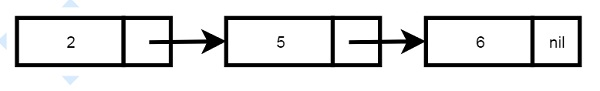Following chapters describe how to generate/create linked lists using recursive structures.

Linked list has two components, the integer part and the link part. The link part will hold another node. End of list will have nil into the link part.In prolog, we can express this using node(2, node(5, node(6, nil))).

Note − The smallest possible list is nil, and every other list will contain nil as the "next" of the end node. In list terminology, the first element is usually called the head of the list, and the rest of the list is called the tail part. Thus the head of the above list is 2, and its tail is the list node(5, node(6, nil)).

We can also insert elements into front and back side −

## Program

```add_front(L,E,NList) :- NList = node(E,L).

NList = node(E,nil).

```

## Output

```| ?- [linked_list].
compiling D:/TP Prolog/Sample_Codes/linked_list.pl for byte code...
D:/TP Prolog/Sample_Codes/linked_list.pl compiled, 7 lines read - 966 bytes written, 14 ms

(15 ms) yes

L1 = node(6,nil)
L2 = node(5,node(6,nil))
L3 = node(2,node(5,node(6,nil)))

yes

L1 = node(6,nil)
L2 = node(6,node(5,nil))
L3 = node(6,node(5,node(2,nil)))

yes

L1 = node(6,nil)
L2 = node(5,node(6,nil))
L3 = node(5,node(6,node(2,nil)))

yes
| ?-
```